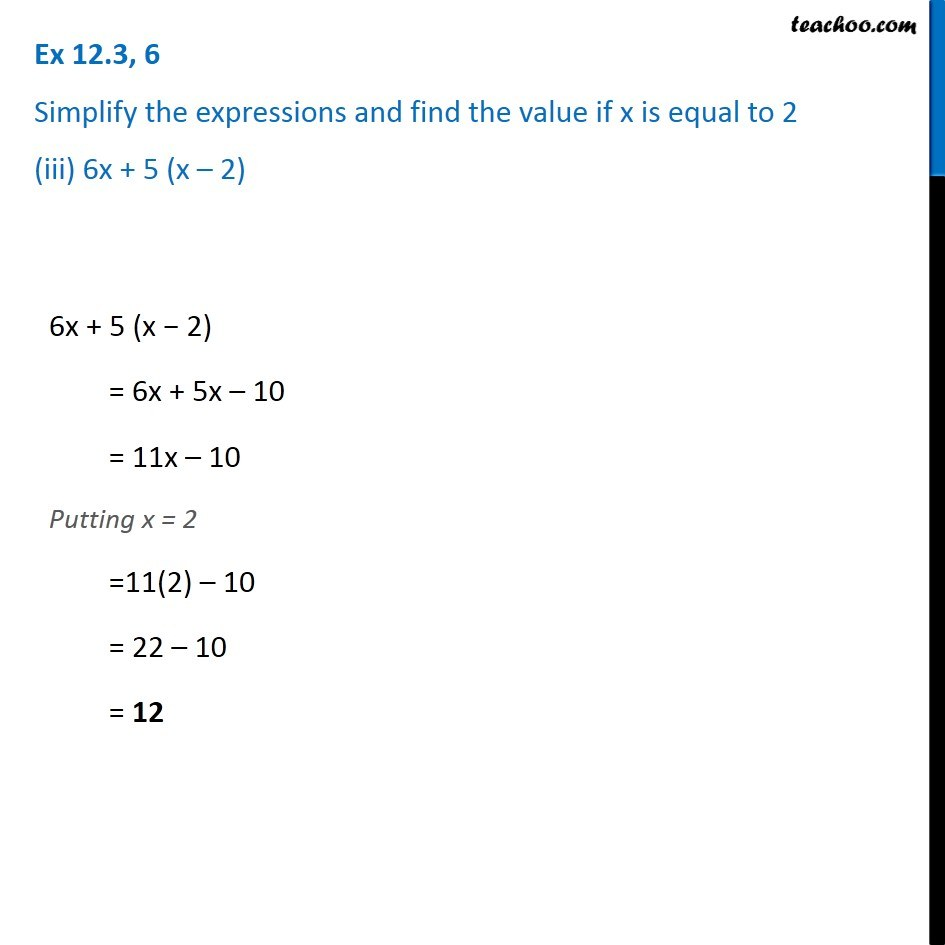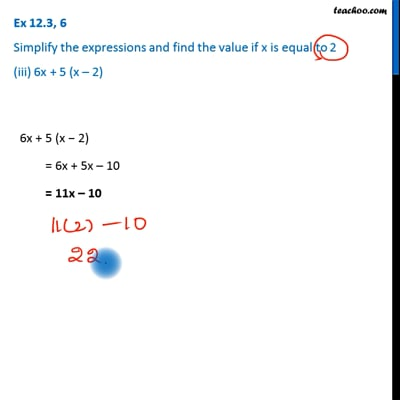Ex 12.3

Chapter 12 Class 7 Algebraic Expressions
Serial order wiseThis video is only available for Teachoo black users

Get live Maths 1-on-1 Classs - Class 6 to 12

### Transcript

Ex 12.3, 6 Simplify the expressions and find the value if x is equal to 2 (iii) 6x + 5 (x – 2) 6x + 5 (x − 2) = 6x + 5x – 10 = 11x – 10 Putting x = 2 =11(2) – 10 = 22 – 10 = 12